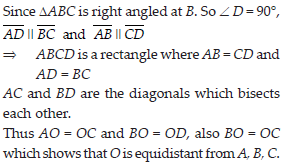Undersatnding Quadrilaterals (Mathematics) Class 8 - NCERT Questions
Q 1.

Given here are some figures.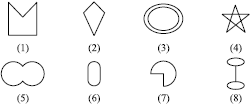Classify each of them on the basis of the following.
(A) Simple curve
(B) Simple closed curve
(C) Polygon
(D) Convex polygon
(E) Concave polygon

SOLUTION: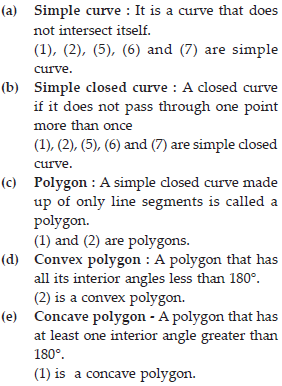Q 2.

How many diagonals does each of the following have?
(B) A regular hexagon
(C) A triangle

SOLUTION:

A diagonal is a line segment joining two non consecutive vertices.
(B) A regular hexagon : A regular hexagon has 9 diagonals.
(C) A triangle : It has 0 diagonal, i.e., no diagonal.

Q 3.

What is the sum of the measures of the angles of a convex quadrilateral? Will this property hold if the quadrilateral is not convex? (Make a non-convex quadrilateral and try!)

SOLUTION: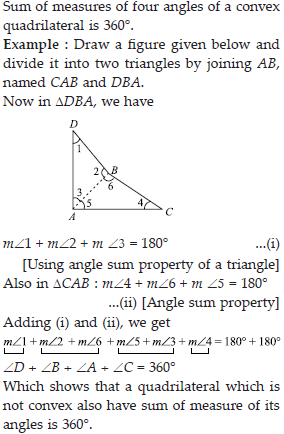Q 4.

Examine the table. (Each figure is divided into triangles and the sum of the angles deduced from that).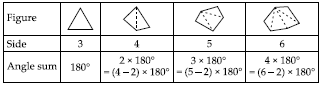What can you say about the angle sum of a convex polygon with number of sides?
(A) 7
(B) 8
(C) 10
(D) n

SOLUTION: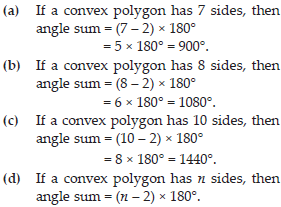Q 5.

What is a regular polygon? State the name of a regular polygon of
(i) 3 sides
(ii) 4 sides
(iii) 6 sides

SOLUTION:

Regular polygon- A polygon, which has all sides of equal length and angles of equal measure is called a regular polygon.
(i) An equilateral triangle, as all 3 sides are equal and all 3 angles are also equal (= 60°).
(ii) A square, as it has all 4 sides equal and all 4 angles are also equal (= 90°).
(iii) A regular hexagon, as it has all 6 sides equal and all 6 angles equal (= 120°).

Q 6.

Find the angle measure x in the following figures.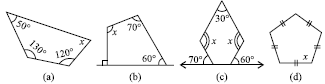SOLUTION: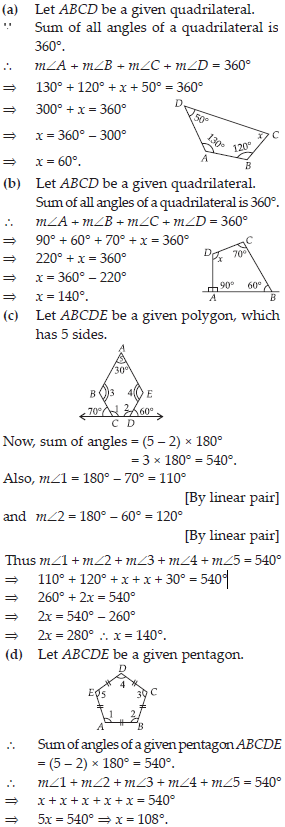Q 7.

(A) Find x + y + z. (B) Find x + y + z + w.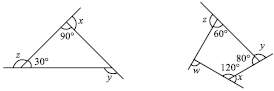SOLUTION: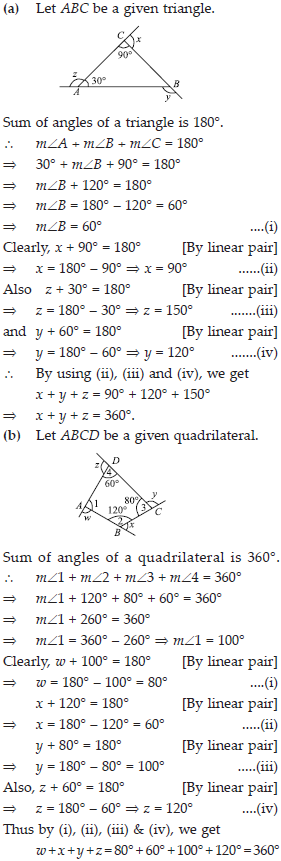Q 8.

Find x in the following figures.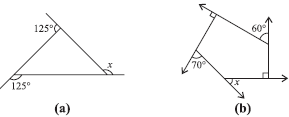SOLUTION: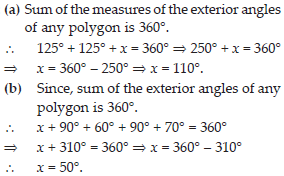Q 9.

Find the measure of each exterior angle of a regular polygon of
(i) 9 sides (ii) 15 sides.

SOLUTION: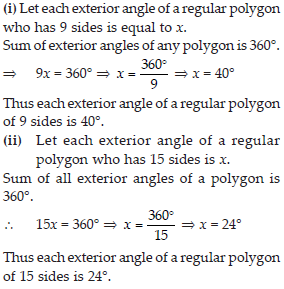Q 10.

How many sides does a regular polygon have if the measure of an exterior angle is 24°?

SOLUTION: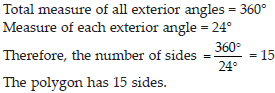Q 11.

How many sides does a regular polygon have if each of its interior angles is 165°?

SOLUTION: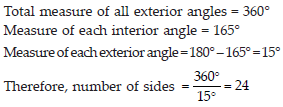Q 12.

(A) Is it possible to have a regular polygon with measure of each exterior angle as 22°?
(B) Can it be an interior angle of a regular polygon? Why ?

SOLUTION: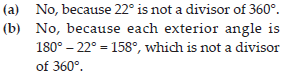Q 13.

(A) What is the minimum interior angle possible for a regular polygon? Why?
(B) What is the maximum exterior angle possible for a regular polygon?

SOLUTION: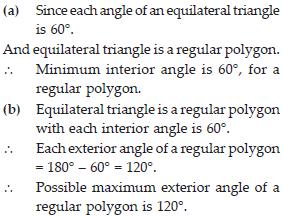Q 14.

Given a parallelogram ABCD. Complete each statement along with the definition or property used.
(ii) ∠DCB = ...........
(iii) OC = ........
(iv) m∠DAB + m∠CDA = ..........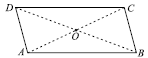SOLUTION: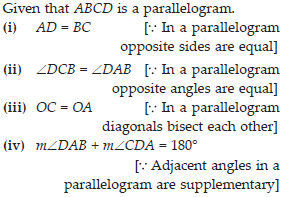Q 15.

Consider the following parallelograms. Find the values of the unknowns x, y, z.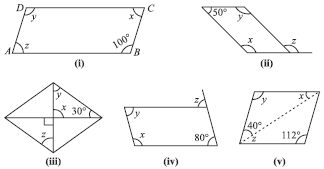SOLUTION: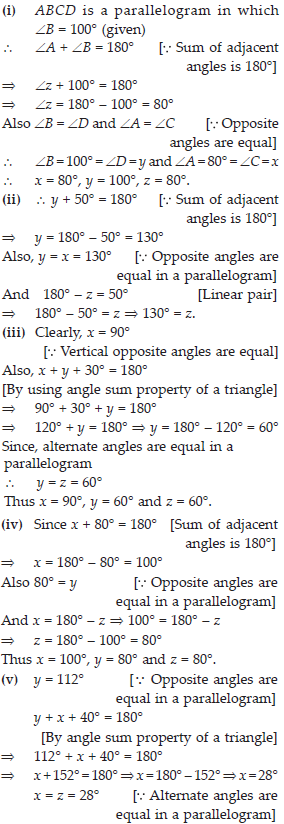Q 16.

Can a quadrilateral ABCD be a parallelogram if
(i) ∠D + ∠B = 180°?
(ii) AB = DC = 8 cm, AD = 4 cm and BC = 4.4 cm?
(iii) ∠A = 70° and ∠C = 65°?

SOLUTION: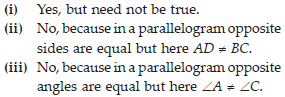Q 17.

Draw a rough figure of a quadrilateral that is not a parallelogram but has exactly two opposite angles of equal measure.

SOLUTION: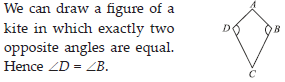Q 18.

The measures of two adjacent angles of a parallelogram are in the ratio 3 : 2. Find the measure of each of the angles of the parallelogram.

SOLUTION: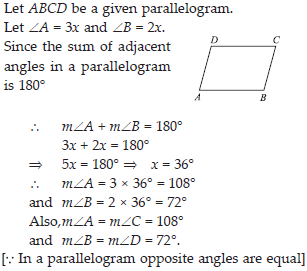Q 19.

Two adjacent angles of a parallelogram have equal measure. Find the measure of each of the angles of the parallelogram.

SOLUTION: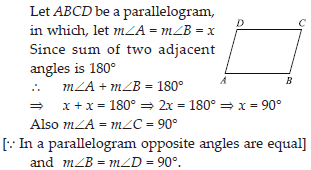Q 20.

The given figure HOPE is a parallelogram. Find the angle measures x, y and z. State the properties you use to find them.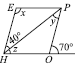SOLUTION: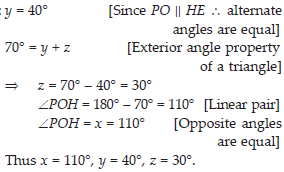Q 21.

The following figures GUNS and RUNS are parallelograms. Find x and y. (Lengths are in cm).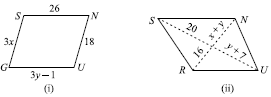SOLUTION: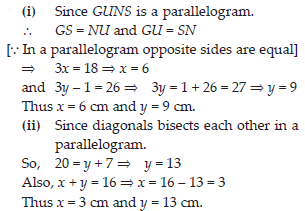Q 22.

In the given figure both RISK and CLUE are parallelograms. Find the value of x.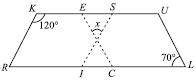SOLUTION: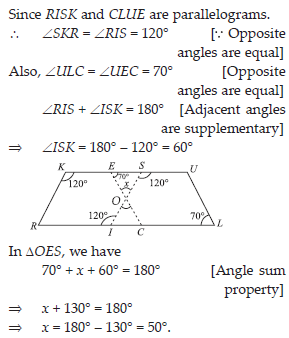Q 23.

Explain how this figure is a trapezium. Which of its two sides are parallel?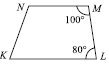SOLUTION:

In a trapezium, only one pair of opposite sides are parallel, whereas other pair of opposite sides are non-parallel.
∴ KLMN is a trapezium because MN || KL. [ Sum of two adjacent interior angles is 180° = ( 80° + 100°)].

Q 24.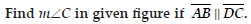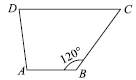SOLUTION: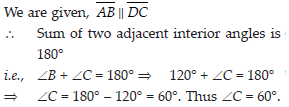Q 25.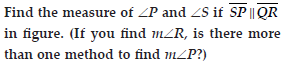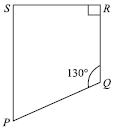SOLUTION: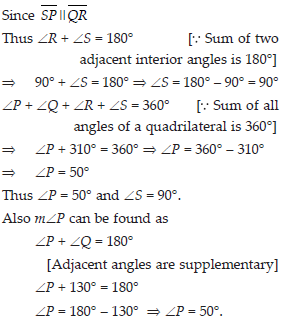Q 26.

State whether True or False.
(A) All rectangles are squares.
(B) All rhombuses are parallelograms.
(C) All squares are rhombuses and also rectangles.
(D) All squares are not parallelograms.
(E) All kites are rhombuses.
(F) All rhombuses are kites.
(G) All parallelograms are trapeziums.
(H) All squares are trapeziums.

SOLUTION:

(a) No, because in a square all sides are equal, but it is not true in case of rectangle.
(b) Yes, because in both diagonals bisects each other and opposite sides are equal.
(c) Yes, because in square all sides are equal. (d) No, all squares are parallelograms. Because all squares satisfies the conditions of a parallelogram.
(e) No, because only two pair of consecutive sides are equal in kites whereas in rhombus all sides are of equal length.
(f) Yes, all rhombuses are kites.
(g) Yes, since both has alteast one pair of parallel sides.
(h) Yes, both has atleast one pair of parallel sides.

Q 27.

Identify all the quadrilaterals that have
(a) four sides of equal length
(b) four right angles

SOLUTION:

(a) The quadrilaterals, those have four sides of equal length are square and rhombus.
(b) The quadrilaterals, those have four right angles, are square and rectangle.

Q 28.

Explain how a square is
(ii) a parallelogram
(iii) a rhombus
(iv) a rectangle

SOLUTION:

(i) A square is four sided, so it is a quadrilateral.
(ii) Since a square has opposite sides parallel and diagonals bisect each other, so it is a parallelogram.
(iii) Since square is a parallelogram with all 4 sides equal, so it is a rhombus.
(iv) Since square is a parallelogram with each angle a right angle, so it is a rectangle.

Q 29.

(i) bisect each other
(ii) are perpendicular bisectors of each other
(iii) are equal

SOLUTION:

(i) The quadrilaterals in which diagonals bisect each other are rhombus, rectangle, square and parallelogram.
(ii) The quadrilaterals in which diagonals are perpendicular bisectors of each other are rhombus and square.
(iii) The quadrilaterals in which diagonals are equal are square and rectangle.

Q 30.

Explain why a rectangle is a convex quadrilateral.

SOLUTION:

When we draw the diagonals joining the end points of a rectangle it lies in its interior. So, it is a convex quadrilateral.

Q 31.

ABC is a right-angled triangle and O is the mid point of the side opposite to the right angle. Explain why O is equidistant from A, B and C. (The dotted lines are drawn additionally to help you).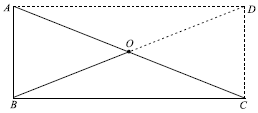SOLUTION: